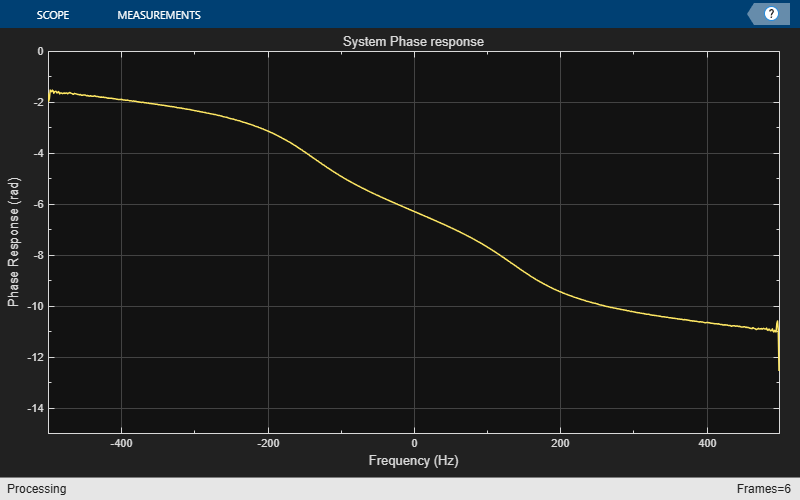# dsp.PhaseExtractor

Extract the unwrapped phase of a complex input

## Description

The `dsp.PhaseExtractor` System object™ extracts the unwrapped phase of a real or a complex input.

To extract the unwrapped phase of a signal input:

1. Create the `dsp.PhaseExtractor` object and set its properties.

2. Call the object with arguments, as if it were a function.

## Creation

### Syntax

``phase = dsp.PhaseExtractor``
``phase = dsp.PhaseExtractor(Name,Value)``

### Description

example

````phase = dsp.PhaseExtractor` returns a phase extractor System object that extracts the unwrapped phase of an input signal.```

example

````phase = dsp.PhaseExtractor(Name,Value)` returns a phase extractor System object with the specified property name set to the specified value.```

## Properties

expand all

Unless otherwise indicated, properties are nontunable, which means you cannot change their values after calling the object. Objects lock when you call them, and the `release` function unlocks them.

If a property is tunable, you can change its value at any time.

Specify if the phase is to be unwrapped only within the frame, as a logical scalar.

When you set this property to:

• `false` –– The object returns the unwrapped phase while ignoring boundaries between input frames.

• `true` –– The object treats each frame of input data independently, and resets the initial cumulative unwrapped phase value to zero each time a new input frame is received.

## Usage

### Syntax

``p = phase(input)``

### Description

example

````p = phase(input)` extracts the unwrapped phase, `p`, of the input signal. Each column of the `input` signal is treated as a separate channel. The System object unwraps the phase of each channel of the input signal independently over time.```

### Input Arguments

expand all

Data input, specified as a vector or a matrix. This object supports variable-size input signals. That is, you can change the input frame size (number of rows) even after calling the algorithm. However, the number of channels (number of columns) must remain constant.

Data Types: `single` | `double`
Complex Number Support: Yes

### Output Arguments

expand all

Unwrapped phase of the input, returned as a vector or a matrix. The size and data type of the unwrapped phase output match the size and data type of the input signal.

Data Types: `single` | `double`

## Object Functions

To use an object function, specify the System object as the first input argument. For example, to release system resources of a System object named `obj`, use this syntax:

`release(obj)`

expand all

 `step` Run System object algorithm `release` Release resources and allow changes to System object property values and input characteristics `reset` Reset internal states of System object

## Examples

collapse all

Create a `dsp.SineWave` System object™. Specify that the object generates an exponential output with a complex exponent.

```sine = dsp.SineWave('Frequency',10,... 'ComplexOutput',true,'SamplesPerFrame',128); ```

Create a `dsp.PhaseExtractor` System object?. Specify that the object ignores frame boundaries when returning the unwrapped phase.

```phase = dsp.PhaseExtractor('TreatFramesIndependently',false); ```

Extract the unwrapped phase of a sine wave. Plot the phase versus time using a `timescope` System object.

```timeplot = timescope('PlotType','Line','SampleRate',1000,... 'TimeSpanSource','Property','TimeSpan',1.5,'YLimits',[0 80],... 'ShowGrid',true,... 'YLabel','Unwrapped Phase (rad)'); for ii = 1:10 sineOutput = sine(); phaseOutput = phase(sineOutput); timeplot(phaseOutput) end ```Create a `dsp.TransferFunctionEstimator` System object™.

```tfe = dsp.TransferFunctionEstimator('FrequencyRange','centered'); ```

Create a `dsp.PhaseExtractor` System object™. Specify that the object must treat each frame of data independently.

```phase = dsp.PhaseExtractor('TreatFramesIndependently',true); ```

Create a `dsp.IIRFilter` System object™. Compute the transfer function of a third-order IIR filter. Use the `butter` function to generate coefficients for the filter.

```[b,a] = butter(3,.3); iir = dsp.IIRFilter('Numerator',b,'Denominator',a); ```

Extract the phase response of the transfer function. Plot using a `dsp.ArrayPlot` System object™.

```sampleRate = 1e3; phaseplot = dsp.ArrayPlot('PlotType','Line','XOffset',-sampleRate/2,... 'YLimits',[-15 0],... 'YLabel','Phase Response (rad)',... 'XLabel','Frequency (Hz)',... 'Title','System Phase response'); for ii = 1:100 % Generate input input = 0.05*randn(1000,1); % Pass through IIR filter filterOutput = iir(input); % Estimate transfer function transferFunction = tfe(input,filterOutput); % Plot transfer function phase phaseOutput = phase(transferFunction); phaseplot(phaseOutput); end ```## Algorithms

Consider an input frame of length N:

`$\left(\begin{array}{l}{x}_{1}\\ {x}_{2}\\ ⋮\\ {x}_{N}\end{array}\right)$`

The object acts on this frame and produces this output:

`$\left(\begin{array}{l}{\Phi }_{1}\\ {\Phi }_{2}\\ ⋮\\ {\Phi }_{N}\end{array}\right)$`

where:

`${\Phi }_{i}={\Phi }_{i-1}+\text{angle}\left({x}_{i-1}^{*}{x}_{i}\right)$`

Here, i runs from 1 to N. The `angle` function returns the phase angle in radians.

If the input signal consists of multiple frames:

• If you set `TreatFramesIndependently` to `true`, the object treats each frame independently. Therefore, in each frame, the object calculates the phase using the preceding formula where:

• ${\Phi }_{0}$ is 0.

• ${x}_{0}$ is 1.

• If you set `TreatFramesIndependently` to `false`, the object ignores boundaries between frames. Therefore, in each frame, the `step` method calculates the phase using the preceding formula where:

• ${\Phi }_{0}$ is the last unwrapped phase from the previous frame.

• ${x}_{0}$ is the last sample from the previous frame.

## Version History

Introduced in R2014b